Next: EM and MAP Up: Ensemble learning Previous: Model selection in ensemble

## Connection to coding

The cost function can be derived within MDL framework. The intuitive idea behind MDL is that in order to be able to encode data compactly one has to find a good model for it. Coding is not actually done; only the formula for the code length is needed. The optimal code length of x is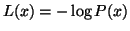bits, and therefore coding has a close connection to the probabilistic framework. Some people prefer to think in terms of coding lengths, some in term of probabilities.

In coding, the sender and the receiver have to agree on the structure of the message in advance. This corresponds to having to state one's priors in the Bayesian framework.

A simple example: let x be the observation to be coded and y be a real valued parameter. We first encode y with accuracy dy. This part of the message takes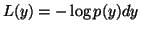bits. Then we encode x with accuracy dx using the value y. This takes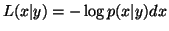bits. The accuracy of the encoding of the observations can be agreed on in advance, but there is a question of how to decide the accuracy dy. The second part of the code would be shortest if y would be exactly in the maximum likelihood solution. If y is encoded in too high accuracy, however, the first part of the code will be very long. If y is encoded in too low accuracy, the first part will be short but deviations from the optimal y will increase the second part of the code.

The bits-back argument, , overcomes the problem by using infinitesimally small dy but picking the y from a distribution q(y) and encoding a secondary message in the choice of y. Since the distribution q(y) is not needed for decoding x, both the sender and the receiver can run the same algorithm for determining q(y) from x. After finding out q(y), the receiver can decode the secondary message which can have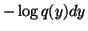bits. As these bits will be got back in the end, the expected message length is

 L(x) =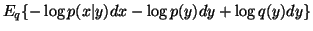=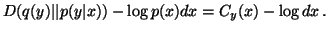(13)

The amount of bits used for individual parameter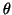can be measured by looking at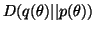It is interesting to notice that although the message has two parts, y and x, if q(y) is chosen to be p(y | x), the expected code length is equal to that of the optimal one-part message where the sender encodes x directly using the marginal probability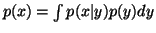.Next: EM and MAP Up: Ensemble learning Previous: Model selection in ensemble
Harri Lappalainen
2000-03-03